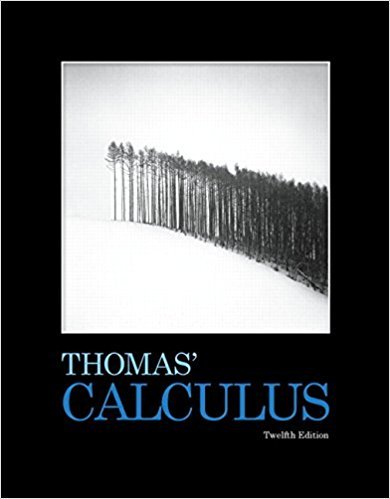×

×

# Solutions for Chapter 15: Multiple Integrals## Full solutions for Thomas' Calculus | 12th Edition

ISBN: 9780321587992Solutions for Chapter 15: Multiple Integrals

Solutions for Chapter 15
4 5 0 262 Reviews
28
5
##### ISBN: 9780321587992

Thomas' Calculus was written by and is associated to the ISBN: 9780321587992. Since 54 problems in chapter 15: Multiple Integrals have been answered, more than 53692 students have viewed full step-by-step solutions from this chapter. Chapter 15: Multiple Integrals includes 54 full step-by-step solutions. This expansive textbook survival guide covers the following chapters and their solutions. This textbook survival guide was created for the textbook: Thomas' Calculus, edition: 12.

Key Calculus Terms and definitions covered in this textbook
• Decreasing on an interval

A function f is decreasing on an interval I if, for any two points in I, a positive change in x results in a negative change in ƒ(x)

• Dot product

The number found when the corresponding components of two vectors are multiplied and then summed

• Equilibrium price

See Equilibrium point.

• Identity matrix

A square matrix with 1’s in the main diagonal and 0’s elsewhere, p. 534.

• Index of summation

See Summation notation.

• Law of sines

sin A a = sin B b = sin C c

• Magnitude of a vector

The magnitude of <a, b> is 2a2 + b2. The magnitude of <a, b, c> is 2a2 + b2 + c2

• Main diagonal

The diagonal from the top left to the bottom right of a square matrix

• Normal curve

The graph of ƒ(x) = e-x2/2

• Order of magnitude (of n)

log n.

• Parametric equations for a line in space

The line through P0(x 0, y0, z 0) in the direction of the nonzero vector v = <a, b, c> has parametric equations x = x 0 + at, y = y 0 + bt, z = z0 + ct.

• Pascal’s triangle

A number pattern in which row n (beginning with n = 02) consists of the coefficients of the expanded form of (a+b)n.

• Positive angle

Angle generated by a counterclockwise rotation.

• Positive association

A relationship between two variables in which higher values of one variable are generally associated with higher values of the other variable, p. 717.

• Standard form of a complex number

a + bi, where a and b are real numbers

• Standard form: equation of a circle

(x - h)2 + (y - k2) = r 2

• Synthetic division

A procedure used to divide a polynomial by a linear factor, x - a

• Vector

An ordered pair <a, b> of real numbers in the plane, or an ordered triple <a, b, c> of real numbers in space. A vector has both magnitude and direction.

• Vertical line

x = a.

• y-axis

Usually the vertical coordinate line in a Cartesian coordinate system with positive direction up, pp. 12, 629.